Perimeter of Triangle

Chapter 10 Class 6 Mensuration
Concept wise

Let’s take a triangle ABC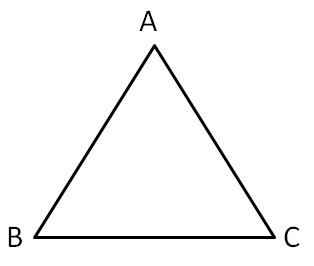Perimeter of ∆ABC = Sum of all sides

= AB + BC + AC

## Perimeter of Isosceles triangle

In an Isosceles triangle,

Two sides are equal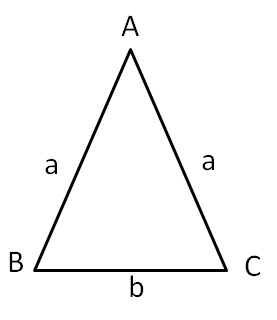Here,

AB = AC = a

BC = b

So,

Perimeter ∆ABC = Sum of all sides

= AB + BC + AC

= a + b + a

= 2a + b

## Perimeter of Equilateral triangle

In an Equilateral triangle,

all sides are eqaul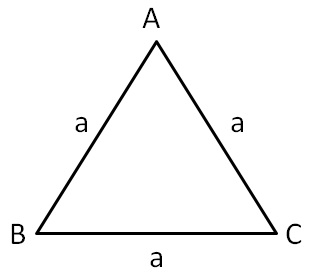Here,

AB = AC = BC = a

So,

Perimeter ∆ABC = Sum of all sides

= AB + BC + AC

= a + a + a

= 3a

Let’s take some examples

## Find perimeter of Δ ABC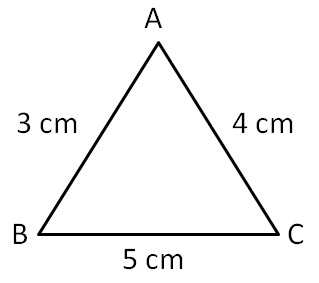Perimeter of ∆ABC = Sum of all sides

= AB + BC + CA

= 3 + 5 + 4

= 12 cm

∴ Required perimeter is 12 cm

## Find perimeter of Δ ABC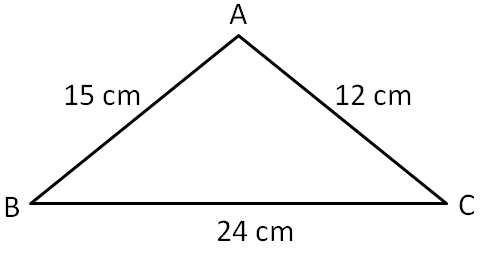Perimeter of ∆ABC = AB + BC + CA

= 15 + 24 + 12

= 51 cm

∴ Required perimeter is 51 cm

## Find perimeter of Δ ABC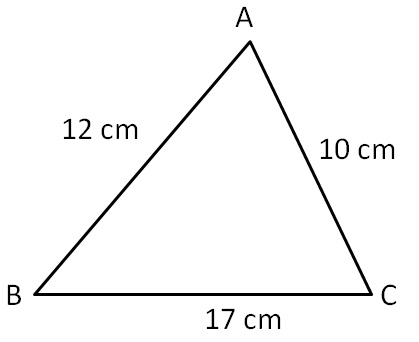Perimeter of ∆ABC = Sum of all sides

= AB + BC + AC

= 12 + 17 + 10

= 39 cm

∴ Required perimeter is 39 cm

## Find perimeter of Δ ABC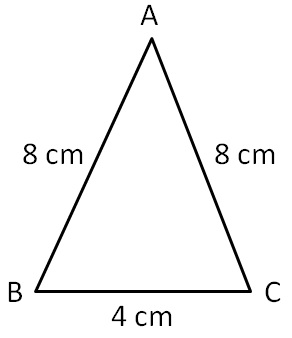Here,

AB = AC = 8cm

BC = 4 cm

Perimeter of ∆ABC = Sum of all sides

= AB + BC + AC

= 8 + 4 + 8

= 20 cm

∴ Required perimeter is 20 cm

## Find perimeter of Δ ABC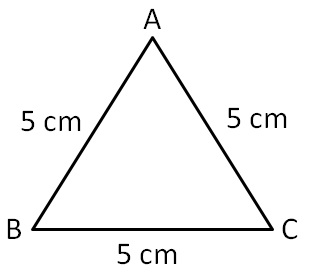Since all sides are equal,

it is an equilateral triangle

Here, AB = BC = CA = a = 5cm

Perimeter of equilateral ∆ABC = 3a

= 3 (5)

= 15 cm

∴ Required perimeter is 15 cm

## Find perimeter of Δ ABC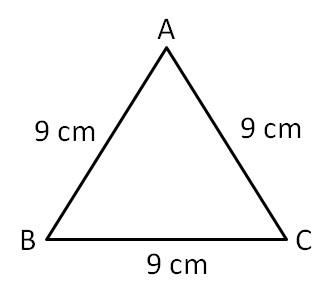Since all sides are equal,

it is an equilateral triangle

Here, AB = BC = CA = a = 9 cm

Perimeter of equilateral ∆ABC = 3a

= 3 (9)

= 27 cm

∴ Required perimeter is 27 cm

Learn in your speed, with individual attention - Teachoo Maths 1-on-1 Class